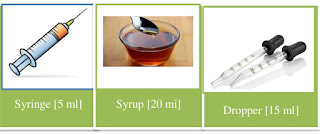# Lesson Planning of Units of Capacity

Lesson Planning of Conversion of Units of Capacity

Subject Mathematics

Students` Learning Outcomes

• Convert liter into millilitres and vice versa.

Information for Teachers

• Capacity can be measured by using millilitres and liter.
• Liter is denoted as L and millilitre as ml.
• The liter was introduced in France in 1795 as “unit of measurement” which is derived from a French unit ‘litron’.
• One liter of liquid water has a mass of almost exactly one kilogram.
• Mill means 1000th part which is used for measuring small quantities.
• 1 liter = 1000 millilitres.
• Liters can be converted into millilitres by multiplying liters with 1000, so, ml = L x 1000.
• Milliners can be converted into liters by dividing millilitre with 1000 so, L = ml/1000.• During this lesson the teacher should consult with textbook at each level wherein and whenever it is required.

Material / Resources

Writing board, chalk/marker, duster, plastic bottles of different sizes, a medicine dropper, water glass, syringe, a cup of tea, syrup, note book and textbook

Introduction

• Ask the students: have you ever noticed how much water your bottle contains?
• Show them label of the bottle that it can hold 1 liter or 1000 millilitre of water in it.
• A cup of tea can hold about 500 ml of tea.
• A glass of water can hold about 250 ml of water in it.

Development

Activity 1

Conversion from Liter to Millilitre:

• Write on the board : as;
• 1 L = 1000 ml
• Show the students plastic bottles with different capacities written on them e.g. ½ litter, 1 liter, 1 ½ litters and 2 ½ litters.
• Ask a student to write capacity of these bottles on the board in a table format and calculate total.
 Students 1 2 3 Total 1.5 L 4.5 L

• Convert these litters in millilitres, as;
• Total capacity of bottles = 4. 5 L
•  1 L  = 1000 ml
• Millilitres   = Liter x 1000
• Total capacity of bottles in millilitres = 4.5 x 1000 = 4500 ml.
• So how many millilitres 1.5 liter contains? ( 1.5 x 1000 = 1500 ml)
• Talk about different items measured in millilitres:

1. Milk
2. Juices
3. Water
4. Syrup

 Activity 2 Conversion from Millilitre to Liter: Show the students different products like syringe, syrup and dropper etc. and mention their capacity on them in ml. Calculate the total capacity of all the products.Tell them by writing on the board that now we will convert these millilitres into liters, as; Total capacity of products = 4 ml 1000 ml = 1 L L = ml/1000 Total capacity of products in liters = 41000 = 0.0041 L

Sum up / Conclusion
• Repeat the key points that you discussed during this lesson.
• Capacity can be measured in liter and millilitres.
• Liter is used to measure capacity of large whereas millilitre to measure small products.
• Mill means 1000th part.
• Liters can be converted into millilitres by multiplying liters with 1000 so, ml = L x 1000 ml.
• Millilitres can be converted into liters by dividing millilitre with 1000 so, L = ml /1000.
• Satisfy the students if still someone have problem in understanding the concept.

Assessment

• Convert the following capacities in the given capacities: as;
• 18. 3 L = ________ml,
• 215 ml = ________L,
• 29 L    = ________ml,
• 33.3 L = ________ml,
• 55 ml  = ________L